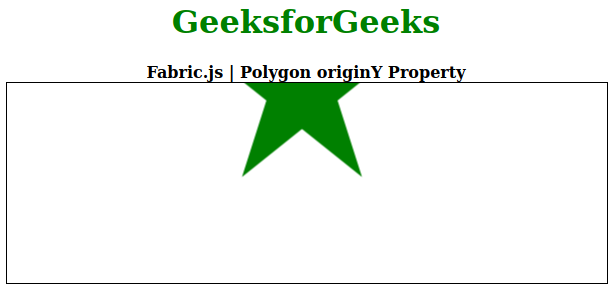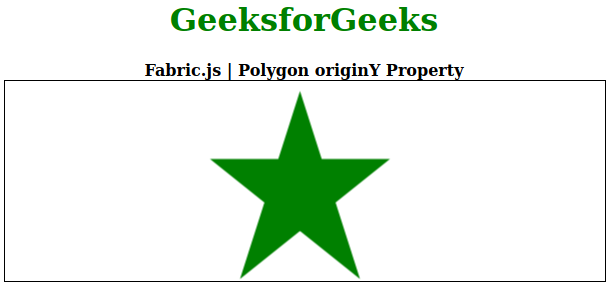Open in App
Not now

# Fabric.js Polygon originY Property

• Last Updated : 26 Aug, 2020

In this article, we are going to see how to set the vertical origin of the transformation of an object canvas polygon using FabricJS. The canvas polygon means the polygon is movable and can be stretched according to requirement. Further, the polygon can be customized when it comes to initial stroke color, height, width, fill color, or stroke width.

To make it possible we are going to use a JavaScript library called FabricJS. After importing the library, we will create a canvas block in the body tag that will contain the polygon. After this, we will initialize instances of Canvas and polygon provided by FabricJS and set the vertical transformation of the polygon using originY property and render the polygon on the Canvas as given in the example below.

Syntax:

```fabric.Polygon([
{ x: pixel, y: pixel },
{ x: pixel, y: pixel },
{ x: pixel, y: pixel},
{ x: pixel, y: pixel},
{ x: pixel, y: pixel }],
{
originY: string
});
```

Parameter: This property accepts a single parameter as mentioned above and described below:

• originY: It specifies the vertical transformation of a canvas polygon.

Note: Dimension pixels is must to create a polygon.

Below examples illustrate the Fabric.JS Polygon originY property in JavaScript:

Example 1:

## HTML

 ` ``<``html``> `` ` `<``head``> ``    ````    ``<``script` `src``= ``"https://cdnjs.cloudflare.com/ajax/libs/fabric.js/3.6.2/fabric.min.js"``> ``    `` `` `` ` `<``body``> ``    ``<``div` `style``=``"text-align: center;width: 600px;"``> ``        ``<``h1` `style``=``"color: green;"``> ``            ``GeeksforGeeks ``        `` ``        ``<``b``> ``            ``Fabric.js | Polygon originY Property ``        `` ``    `` `` ` `    ``<``canvas` `id``=``"canvas"``            ``width``=``"600"``            ``height``=``"200"``            ``style``=``"border:1px solid #000000;"``> ``    `` `` ` `    ``<``script``> `` ` `        ``// Initiate a Canvas instance ``        ``var canvas = new fabric.Canvas("canvas"); `` ` `        ``// Initiate a polygon instance ``        ``var polygon = new fabric.Polygon([ ``        ``{ x: 295, y: 10 }, ``        ``{ x: 235, y: 198 }, ``        ``{ x: 385, y: 78}, ``        ``{ x: 205, y: 78}, ``        ``{ x: 355, y: 198 }], { ``            ``fill: 'green',``            ``originY: 'down'``        ``}); `` ` `        ``// Render the polygon in canvas ``        ``canvas.add(polygon); ``    `` `` `` ` ``

Output:Example 2:

## HTML

 ` ``<``html``> `` ` `<``head``> ``    ````    ``<``script` `src``= ``"https://cdnjs.cloudflare.com/ajax/libs/fabric.js/3.6.2/fabric.min.js"``> ``    `` `` `` ` `<``body``> ``    ``<``div` `style``=``"text-align: center;width: 600px;"``> ``        ``<``h1` `style``=``"color: green;"``> ``            ``GeeksforGeeks ``        `` ``        ``<``b``> ``            ``Fabric.js | Polygon originY Property ``        `` ``    `` `` ` `    ``<``canvas` `id``=``"canvas"``            ``width``=``"600"``            ``height``=``"200"``            ``style``=``"border:1px solid #000000;"``> ``    `` `` ` `    ``<``script``> `` ` `        ``// Initiate a Canvas instance ``        ``var canvas = new fabric.Canvas("canvas"); `` ` `        ``// Initiate a polygon instance ``        ``var polygon = new fabric.Polygon([ ``        ``{ x: 295, y: 10 }, ``        ``{ x: 235, y: 198 }, ``        ``{ x: 385, y: 78}, ``        ``{ x: 205, y: 78}, ``        ``{ x: 355, y: 198 }], { ``            ``fill: 'green',``            ``originY: 'center'``        ``}); `` ` `        ``// Render the polygon in canvas ``        ``canvas.add(polygon); ``    `` `` `` ` ``

Output:My Personal Notes arrow_drop_up